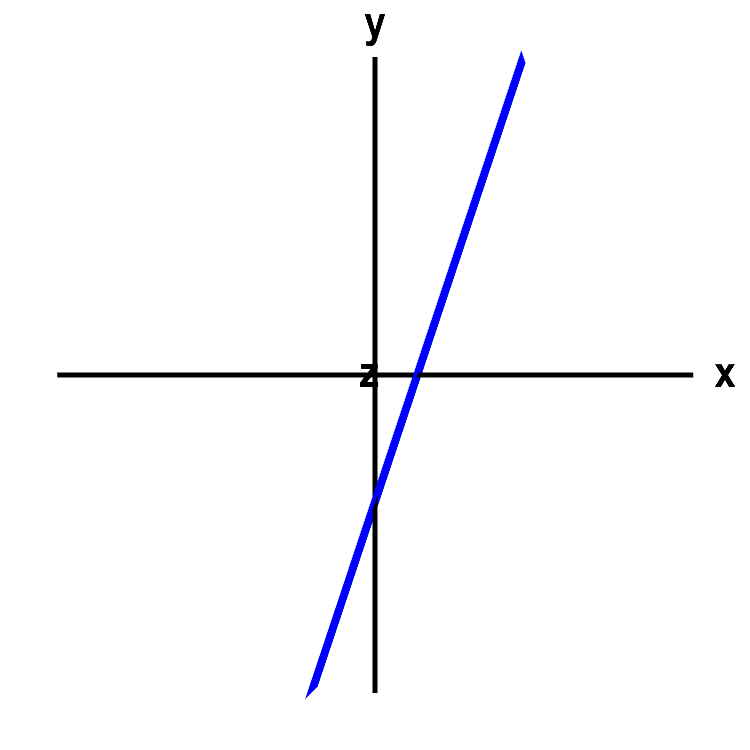# Math Insight

### Applet: An angled line or a planeThe graph of the equation $y=3x-2$ looks like a line, which it would be if were an equation in two dimensions, i.e., in the $xy$-plane. However, rotate the graph with the mouse to give you a new perspective on the graph. Since in this case, the graph is really in three dimensions, the graph of the equation becomes a plane. The equation just happens to not depend on the variable $z$, so the plane is parallel to the $z$-axis.